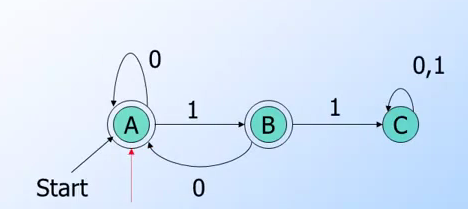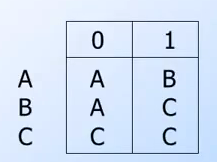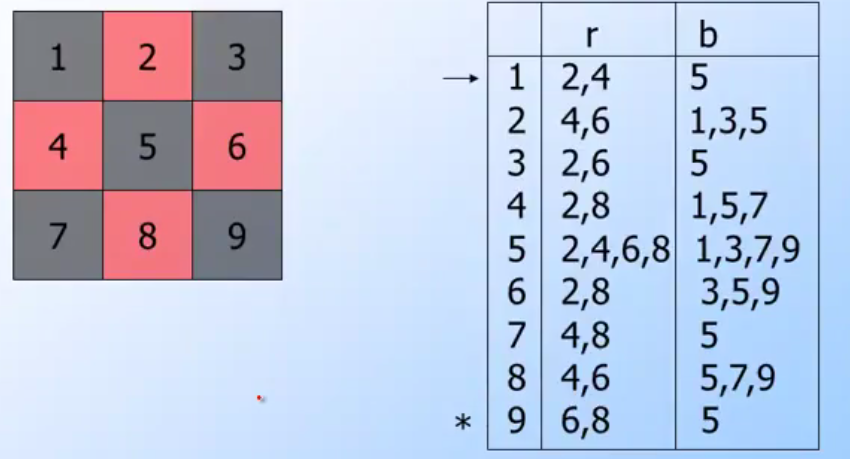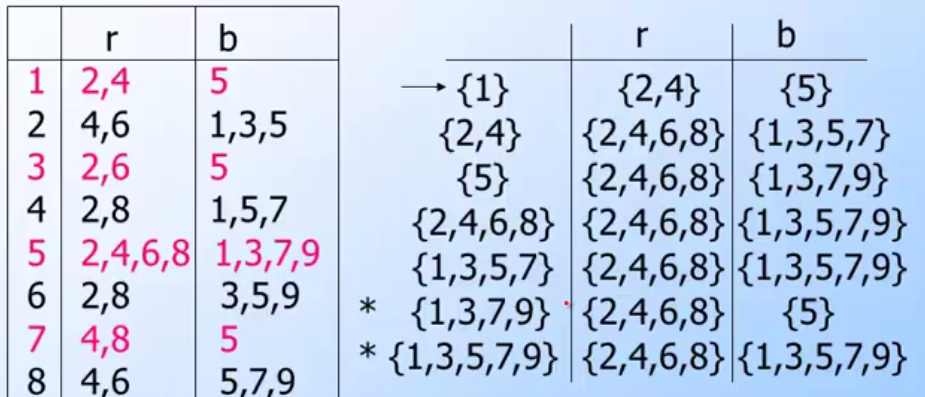## 第一週課程

### DFA(Deterministic Finite Automata)基本定義

• alphabet:
• 任何有限的符號集合． (可以是ASCII也可以是Unicode)
• ex: {0,1} (binary alphabet), {a,b} {s,p} 都是alphabet
• string:
• 透過alphabet產生的list，其中每一個元素都要是該alphabet其中一個元素．
• length代表的是該string有的個數．
• ex alphabet {0, 1} 其 string {01} length=2
• ε 代表是empty string其中他的length=0
• language:
• alphabedt產生出所有string的subset．
• DFA: Deterministic Finite Automata: A formalism for defining languages．由以下組成:
• 有限的狀態(states) 表示為 Q
• 固定的input alphabet 表示為 Σ
• 一個起始狀態 (start state) 表示為q0
• 至少一個結束狀態 (final state) (這裏指的final state也是指可接受的狀態) 表示為 F
• 一個transition function 表示為 δ(Q, A)其中 A 代表是一個輸入的 alphabet
• transition function代表的是，一個輸入所要進入的一個狀態．
• δ(Q, A) 代表是從狀態Q透過輸入A來計算下一個狀態會是哪裡．

{0, 1} 是一個alphabet

{0, 1}* = {ε, 0, 1, 00, 01, 10, 11, 010, …} 是alphabet {0,1}為可能出現的string組合，其中

• {e} length=0
• {0} length=1
• {00} {01} {10} {11} length=2(pic: from coursera automata course lecture)

• 輸入alphabet為{0, 1}
• final state為 B
• dead state為 C (dead node: 也就是只走到C後不論怎麼輸入都無法到達final note)
• start state為 A
• transition function可以表示以下方式: A -> δ(B, 1) -> δ(C, 1)(pic: from coursera automata course lecture)

#### Transition Table 簡單範例

• 在A狀態輸入0會回到A狀態，輸入1會到B狀態．
• 狀態A輸入1, 1 會到狀態C。
##### Transitio Function 運算範例:
• δ(B, 011) -> δ(δ(B, 01), 1) -> δ(δ(δ(B, 0), 1), 1) -> δ(δ(A, 1), 1) -> δ(B, 1) -> C
• δ(A, 0) -> A

### NFA(Nondeterministic Finite Automata)基本定義

NFA跟DFA有些許的不同，這裡先列出幾個幾本定義

• NFA中，可以同時存在多個狀態中，也就是說δ(Q1, A)有可能出現不僅僅一個狀態Q2，而是δ(Q1, A)-> {Q2, Q3}．這代表這裡有路可以從Q1透過路線A可以到達Q2跟Q3
• 跟DFA一樣，NFA一樣開始在一個start state
• 跟DFA一樣，NFA一樣結束在一個以上的final state(pic: from coursera automata course lecture)

    NFA 表現起來，比較像是棋盤式．每一個狀態(格子)同一個輸入有不僅僅一個可以走得下一步(狀態)


#### 如何把NFA轉化成DFA

• DFA: 每一次transition function會到唯一一個狀態，也就是: δ(Q1, A)-> Q2
• NFA: 每一個transition function不一定只會指向一個狀態，所以: δ(Q1, A)-> {Q2, Q3, ...}

    δD(Q1, A) -> P
δN(Q1, A) -> {P} 可以視為 P 為 所有輸出集合的其中一個


    δD(Q1, A) -> P     //一般習慣的DFA表示方式
δN(Q1, A) -> {P}   //一般習慣的NFA表示方式


#### Subset construction

δD({q1, q2, q3, ... qk}, A) 為一個將NFA轉換成DFA的transition function，而結果就是將所有結果的聯集合(union) i = 1, 2, 3 … k是 δN(qi, A)

    δD({q1, q2, q3, ... qk}, A) = union(δN(qi, A))  其中 i = 1, 2, 3 ... k


#### Subset construction的範例• 首先走到state {1} 這時候會透過 r 跟 b 走到 2,4 跟5 ． 由於我們要取每個結果的聯集．所以 {2,4} {5} 會被當作是我們的一個State
• 接下來先走到 {2,4}, 這時候要查看 {2} 與 {4}的結果，也就是 將 {4,6}{2,8}做聯集{2,4,6,8}為r的結果，而將{1,3,5}跟{1,5,7}做聯集{1,3,5,7}．
• 並且將以上兩個結果當作新的state加入之後要走的路途
• 接下來走 {1,3,5,7} 於是又繼續看 {1} {3} {5} {7}的結果．以此類推….### ε-NFA 的基本定義(pic: from coursera automata course lecture)

    每一個NFA都是ε-NFA


#### Example of Closure Starte

• CL(A) = {A} 因為沒有任何ε 的輸入可以到達的狀態．
• CL(B) = {B, D} ，這裡δN(B, ε) -> D
• CL(E) = {B, C, D, E}

#### 更多在ε-NFA關於transition function 的運算

• δ(q, w)在NFA中代表由狀態q開始透過輸入w，可以到達的狀態集合．
• δ(q, ε) = CL(q) 也就是說由狀態q 在沒有輸入(也就是說輸入為空集合ε)可以到達的狀態．
• δ(q, A) = δ(q, Aε) = δ(δ(q, A), ε) 所以可以推導出以下
• δ(q, A) = δ(δ(q, A), ε)) = CL(δ(q, A)) = CL({E}) (假設δ(q, A)={E})